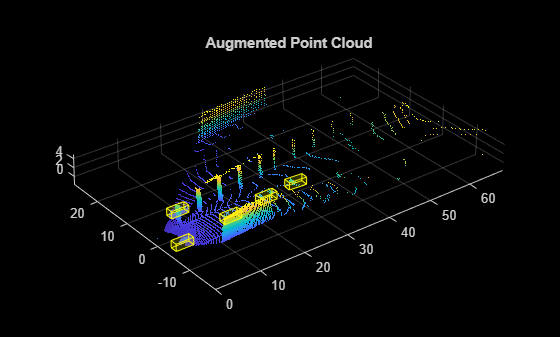# sampleLidarData

Sample 3-D bounding boxes and corresponding points from training data

## Syntax

``[pcds,blds] = sampleLidarData(trainingData,classNames)``
``[pcds,blds] = sampleLidarData(___,Name=Value)``

## Description

example

````[pcds,blds] = sampleLidarData(trainingData,classNames)` samples 3-D bounding boxes and the corresponding points from the specified training data and returns them as datastore objects.```
````[pcds,blds] = sampleLidarData(___,Name=Value)` specifies one or more name-value arguments in addition to all input arguments from the previous syntax. For example, `sampleLidarData(trainingData,classNames,MinPoints=20)` samples only boxes that have a minimum of 20 points inside them.```

## Examples

collapse all

Load a point cloud and its class labels into the workspace.

```unzip("sampleWPIPointClouds.zip",tempdir); dataLocation = fullfile(tempdir,"sampleWPIPointClouds"); load("sampleWPILabels.mat","trainLabels");```

Create a datastore for training data.

```pcds = fileDatastore(dataLocation,"ReadFcn",@(x) pcread(x)); blds = boxLabelDatastore(trainLabels); trainingData = combine(pcds,blds);```

Define the class names to sample from the input data. Use the `sampleLidarData` function to sample the corresponding bounding boxes.

```classNames = {'car'}; [pcdsSampled,bldsSampled] = sampleLidarData(trainingData,classNames,Verbose=false); cdsSampled = combine(pcdsSampled,bldsSampled);```

Read a point cloud from the training data.

```pcBoxLabels = read(trainingData); figure pcshow(pcBoxLabels{1,1}.Location) showShape(cuboid=pcBoxLabels{1,2},Opacity=0.1, ... Color="yellow",LineWidth=0.5); title("Original Point Cloud")```Augment the point cloud data `pcBoxLabels` with points sampled from the datastore `cdsSampled` using the `pcBboxOversample` function.

```totalObjects = 5; augmentedPcBoxLabels = pcBboxOversample(pcBoxLabels,cdsSampled,classNames,totalObjects); figure pcshow(augmentedPcBoxLabels{1,1}.Location) showShape(cuboid=augmentedPcBoxLabels{1,2},Opacity=0.1, ... Color="yellow",LineWidth=0.5); title("Augmented Point Cloud")```## Input Arguments

collapse all

Input point cloud data, specified as a valid datastore object or a table.

• If you specify a datastore object, your data must be set up such that using the `read` function on the datastore object returns a cell array or table with three columns. Each row corresponds to a point cloud, and the columns must follow this format.

• First column — Organized or unorganized point cloud data, specified as a `pointCloud` object.

• Second column — Bounding boxes, specified as a cell array containing an M-by-9 matrix. Each row of the matrix is of the form [x y z length width height roll pitch yaw], representing the location and dimension of a bounding box. M is the number of bounding boxes.

• Third column — Labels, specified as a cell array containing an M-by-1 categorical vector containing the object class names.

You can use the `combine` function to combine two or more datastores. For more information on creating datastore objects, see the `datastore` function.

• If you specify a table, the table must have two or more columns. The first column must contain point cloud file names. The point cloud files can be in any format supported by the `pcread` function. Each of the remaining columns contains a cell array that represents a single object class, such as `Car`, or `Truck`. Each cell contains an M-by-9 matrix. Each row of the matrix is of the form [x y z length width height roll pitch yaw], specifying the location and dimensions of the bounding box in the corresponding point cloud. M is the number of bounding boxes.

Name of the object classes, specified as a character vector, string scalar, vector of strings, or a cell array of character vectors. The function samples these classes from the input training data. For example, 'car', 'truck', or 'pedestrian'.

### Name-Value Arguments

Specify optional pairs of arguments as `Name1=Value1,...,NameN=ValueN`, where `Name` is the argument name and `Value` is the corresponding value. Name-value arguments must appear after other arguments, but the order of the pairs does not matter.

Example: `sampleLidarData(trainingData,classNames,MinPoints=20)` samples only objects that have a minimum of 20 points inside them.

Minimum number of points required to sample an object, specified as a positive scalar or an M-element vector. M is the number of classes. If the value is a vector, each element corresponds to the respective class. Otherwise the function uses the same value for all the classes.

Data Types: `single` | `double` | `int8` | `int16` | `int32` | `int64` | `uint8` | `uint16` | `uint32` | `uint64`

Folder in which to write the sampled data, specified as a character vector or string scalar. The folder must exist in the location specified, and you must have write permissions. By default, the function writes this data into the current working folder. The data consists of the sampled points and their respective box labels.

Data Types: `char` | `string`

Display of data writing progress, specified as a logical `true` or `false`.

Data Types: `logical`

## Output Arguments

collapse all

File locations of the points sampled from the training data, returned as a `fileDatastore` object.

Sampled 3-D bounding boxes and labels, returned as a `boxLabelDatastore` object.

## Algorithms

Lidar object detection techniques directly predict 3-D bounding boxes around objects of interest. Data augmentation helps you improve prediction accuracy and avoid overfitting issues while training.

You can perform ground truth data augmentation on point clouds using these steps.

1. Sample 3-D bounding boxes and the corresponding points from input training data using the `sampleLidarData` function.

2. Augment a point cloud randomly with the sampled bounding boxes by using the `pcBboxOversample` function. The function performs a collision test on the sampled boxes and the ground truth boxes of the input point cloud to avoid overlap.

This technique alleviates the class imbalance problem in lidar object detection.

## Version History

Introduced in R2022a